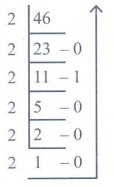Home | | Computer Science 11th std | Answer the following questions

## Chapter: 11th Computer Science : Chapter 2 : Number Systems

Short Answers, Explain in Brief, Explain in detail, Important Questions - Computer Science : Number Systems

Fundamentals of Computers

Number Systems

Evaluation

1. What is data?

Answer: The term data comes from the word datum, which means a raw fact. The data is a fact about people, places or some objects.

2. Write the 1’s complement procedure.

Step 1: Convert given Decimal number into Binary

Step 2: Check if the binary number contains 8 bits, if less add 0 at the left most bit, to make it as 8 bits.

Step 3: Invert all bits (i.e. Change 1 as 0 and 0 as 1).

3. Convert (46)10 into Binary number4610 = (101110)2

4. We cannot find 1’s complement for (28)10. State reason.

Answer: (28)10 is positive number. 1’s Complement represent signed numbers (Negative numbers) only. So, (28)10 cannot find 1’s complement.

5. List the encoding systems for characters in memory.

Answer: (i) BCD - Binary Coded Decimal.

(ii) EBCDIC - Extended Binary Coded Decimal Interchange Code.

(iii) ASCII - American Standard Code for Information Interchange.

(iv) Unicode.

(v) ISCII - Indian Standard Code for Information Interchange.

1. What is radix of a number system? Give example

Answer: A numbering system is a way of representing numbers. Each number system is uniquely identified by its base value or radix. Radix or base is the count of number of digits in each number system. Radix or base is the general idea behind positional numbering system.

Example :

Binary Number System - Radix 2

Octal Number System - Radix 8

Decimal Number System - Radix 10

2. Write note on binary number system.

Answer: (i) There are only two digits in the Binary system, namely, 0 and 1. The numbers in the binary system are represented to the base 2 and the positional multipliers are the powers of 2.

(ii) The left most bit in the binary number is called as the Most Significant Bit (MSB) and it has the largest positional weight.

(iii) The right most bit is the Least Significant Bit (LSB) and has the smallest positional weight.

3. Convert (150)10 into Binary, then convert that Binary number to Octal

15010 = ?100101102 = 2268.

4. Write short note on ISCII

Answer: (i) ISCII is the system of handling the character of Indian local languages. This as a 8-bit coding system.

(ii) Therefore it can handle 256 (28) characters. This system is formulated by the department of Electronics in India in the year 1986-88 and recognized by Bureau of Indian Standards (BIS).

(iii) Now this coding system is integrated with Unicode.

5. Add a) -2210+1510 b) 2010+2510

(a) −2210 + 1510(1111)2  (00001111)2

(10110)2  (00010110)2

1’s complement = 11101001

2's complement = 11101010

(−22)10 + (15)10 = 11101001

= 00001111

==========

11111001

==========

(b) 2010 + 25102010 + 2510 = 001011012

1. a) Write the procedure to convert fractional Decimal to Binary

b) Convert (98.46)10 to Binary

a) The method of repeated multiplication by 2 has to be used to convert such kind of decimal fractions. The steps involved in the method of repeated multiplication by 2:

Step 1: Multiply the decimal fraction by 2 and note the integer part. The integer part is either 0 or 1.

Step 2: Discard the integer part of the previous product. Multiply the fractional part of the previous product by 2. Repeat Step 1 until the same fraction repeats or terminates (0).

Step 3: The resulting integer part forms a sequence of 0s and 1s that become the binary equivalent of decimal fraction.

Step 4: The final answer is to be written from first integer part obtained till the last integer part obtained.

(b) 98.4610

1. Integer part2. Fractional part

0.46 × 2 = 0.92 = 0

0.92 × 2 = 1.84 = 1

0.84 × 2 = 1.68 = 1

0.68 × 2 = 1.36 = 1

0.36 × 2 = 0.72 = 0

0.72 × 2 = 1.44 = 1

98.4610 = (1100010.011101....)2

2. Find 1’s Complement and 2’s Complement for the following Decimal number

a) -98 b) -135

a) -98b) -135b) Subtract 11010112 - 1110102